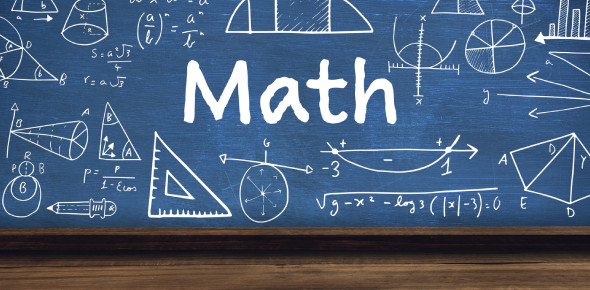# SAT Math Questions: Quiz! Trivia

24 Questions | Attempts: 34146Settings.

• 1.
If A \$ B = A * B - ( A + B), what is 3 \$ (2 \$ 1)?
• A.

-5

• B.

-3

• C.

0

• D.

1

• E.

4

• 2.
How many numbers less than 1000 are divisble by 3?
• A.

300

• B.

310

• C.

311

• D.

333

• E.

500

• 3.
If a^b = c^d, which of the following is not necessarily true?
• A.

A^b - c^d = 0

• B.

A^b + c^d = 2 * (a^b)

• C.

(a^b)/(c^d) = 1

• D.

A = c

• E.

A^b * c^d = a^(2*b)

• 4.
Which of the following lines does not intersect y = 5 x + 2?
• A.

-5x + 2y = 4

• B.

-2x + 5y = -3

• C.

10x - y = 1

• D.

3x + y = 17

• E.

5x - y = -29

• 5.
A regular polygon has 9 sides. What is the degree measure of the angle, within the polygon, between any two sides?
• A.

60

• B.

90

• C.

120

• D.

140

• E.

165

• 6.
A cubic box, X, has sides of length n. Another cubic box, Y, has sides of length 2n. How many boxes X could fit into a single box Y?
• A.

2

• B.

4

• C.

8

• D.

16

• E.

32

• 7.
A number is called "round" if it contains at least one zero as a digit. How many three-digit numbers are "round?"
• A.

153

• B.

171

• C.

178

• D.

179

• E.

215

• 8.
How many ways can Pete, Mary, Sue, and Joe stand in a line if Joe and Sue cannot stand next to each other?
• A.

4

• B.

6

• C.

12

• D.

16

• E.

18

• 9.
A square, X, has sides of length n. Another square, Y, has sides of length 1.5n. How many X can fit into a single Y?
• A.

1

• B.

1.5

• C.

2

• D.

2.25

• E.

4

• 10.
A triangle has sides of length 7, 11, and X. Which of the following cannot be X?
• A.

2

• B.

4

• C.

8

• D.

12

• E.

18

• 11.
If |a| < |b|, and a > b, which of the following is necessarily true?
• A.

|a + b| > |b| + |a|

• B.

|a + b| < a - b

• C.

|a| + |b| > 2|b|

• D.

|a - b| > a + b

• E.

|a| - |b| > |a - b|

• 12.
Six children sit at a circular table. In how many orders can they sit at the table?
• A.

6

• B.

18

• C.

64

• D.

118

• E.

120

• 13.
If a two-sided coin is flipped three times, what is the probability that at least one head will show up?
• A.

1/8

• B.

3/8

• C.

1/2

• D.

2/3

• E.

7/8

• 14.
If m & n = (m + n)^(m - n), what is 2 & (2 & 2)?
• A.

2

• B.

3

• C.

4

• D.

6

• E.

8

• 15.
Which of the following cannot be formed from any combination of two pennies, three nickles, one dime, and two quarters?
• A.

\$0.03

• B.

\$0.54

• C.

\$0.56

• D.

\$0.75

• E.

\$0.78

• 16.
A three-digit number is called "big" if any two of its digits are equal. How many three-digit numbers are "big?"
• A.

112

• B.

146

• C.

214

• D.

252

• E.

316

• 17.
If 2 ^ (4x + 3) = 4 ^ (x - 1), what is x?
• A.

-3

• B.

-5/2

• C.

-1

• D.

2

• E.

4/3

• 18.
Which of the following values of x is not in the domain of the function y = x / (x^2-2x+1)
• A.

-3

• B.

-2

• C.

-1

• D.

0

• E.

1

• 19.
If a + b = y, what is a^2 + 2ab + b^2?
• A.

Y

• B.

2y

• C.

Y^2

• D.

2y^2

• E.

4y^2

• 20.
The number 100 has two trailing zeros. How many trailing zeros does 100! have?
• A.

12

• B.

15

• C.

18

• D.

24

• E.

28

• 21.
Which of the following statements is always true?
• A.

|(a+b)^2| < |a^2| + |b^2|

• B.

|a^2 + b^2| > (a+b)^2

• C.

|a^2 + b^2| >= |a+b|^2

• D.

|a^2 + b^2 - 1|

• E.

|a + b^2| > |a^2 - b|

• 22.
In physics, force = mass * acceleration. Suppose you have an original force F and new force G in which the mass is increased by a factor of two and the acceleration is increased by a factor of four. What is the ratio of G:F?
• A.

1:8

• B.

1:4

• C.

1:1

• D.

4:1

• E.

8:1

• 23.
If a^2 = b^2, which of the following is/are always true? I. a = b II. |a| = |b| III. |a - b| = 0
• A.

I only

• B.

II only

• C.

I and II

• D.

I and III

• E.

I, II, and III

• 24.
If (a-b)^2 = (a+b)^2, what is the value of ab?
• A.

-4

• B.

-2

• C.

0

• D.

2

• E.

4

## Related TopicsBack to top
×

Wait!
Here's an interesting quiz for you.